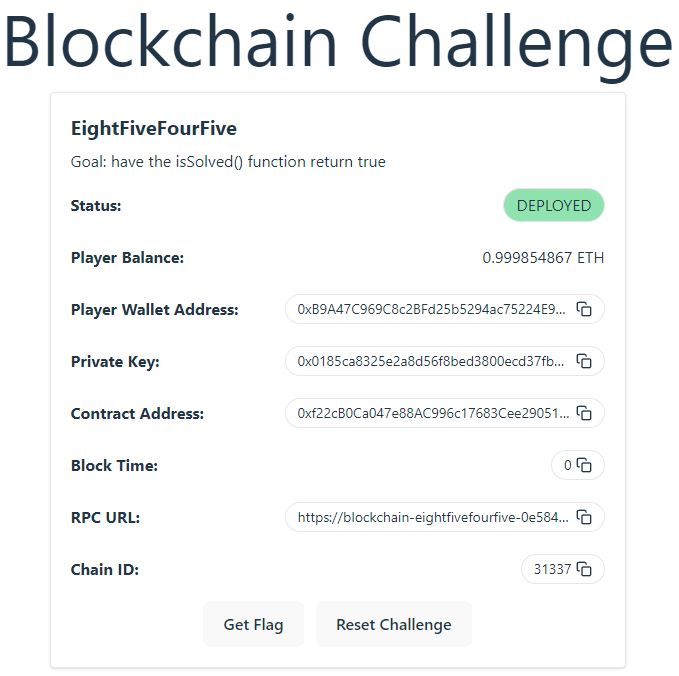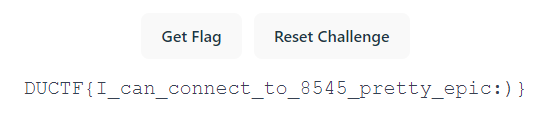# DUCTF 2023 Writeups

## August 31, 2023

This weekend I played DownUnderCTF 2023. The fourth instalment of the now huge DownunderCTF. This round they provided a new category of beginner challenges all grouped together. I did the majority of these as well as some other challenges.

These are my solutions for the challenges `flag art` and `eight five four five`.

#### flag art - beginner crypto - 100 points

This beginner challenge was a fun one, mainly because encoding a message in ASCII art was pretty fun. The files provided for this one were `output.txt` and `flag-art.py` which was the script that generated the output.

The script was:

``````message = open('./message.txt', 'rb').read() + open('./flag.txt', 'rb').read()

#print(f"message len: {len(message)}")

palette = '.=w-o^*'

canvas = ''
for c in message:
for m in [2, 3, 5, 7]:
while True:
t = template.pop(0)
if t == 'X':
canvas += palette[c % m]
break
else:
canvas += t

print(canvas)
``````

Which generated:

``````                                                           ==
wo=.=*.w.        ^==-
^..ow==w*.w=o=        .w^.
.--==w*.w=o=...=.=         *.w.^==
.-.wwo=.=*.w.^.wwo==.-=.=     *..--.=-*=
....w.^==-^.wwo.w=o.wo*=...==.-.wwo=.o=.wo
*==w*..--..--=w=-.w.^==-^=w=-=.-^.wwo..o..wo*
=w=-.wwo==oo==w*==.-=www.wwo.wo*==w*==.-.wwo=.w=..-
*..-*.wwo.=-o=.oo==.-.wwo==.-      =.=*        .wwo.wo*=w=-..
ow=w=-.wwo==w*..ow=w=-.wwo                       ==.-=.=*==oo=w=-.
wwo..ow==w*.w.^.=.w=.=*==o                       o.wwo=wo.=.=*..ow.=
.w==.-.wwo.w=o=.=*.wwo==oo                       ==w*=www=w=-.wwo.w=o.w
o*=w=-.wwo==oo=w=-==.-==.-                       ==w*==-^=w=-.wwo..--=.
=*.w.^==-^.wwo=w=-.w.^=.=*      =.w^      ==-^   .wo*.==o.wwo=wo^=.=*=.
w^..ow.wwo..wo..--==w*==-^.wwo=...==.-.=-w.w   wo.w-^==.===wo..o..=..
=.-o..ow=.=w=.o=..-*.w.^==.-.w=o..ow=.w^=.   o=.w=o==o...-*.w.^=w.
o..-*..wo=w.o..wo..--.=w-==-^=w.o..wo..ow.   .-*==oo=w.o..wo..--.
=w-==-^=w.o.=w-..ow==.        *=w.o.w-.===   w=w.o..--..-*..-*=
www=.w^.=w.=w.o.w=              o.=w-.w-...--=.=w=w.o..-*..ow
=w.o=                             .o=.wo*==o..w.^=.=w==.
-=.=w=w.o..ow=.=w==
oo.=w-==o..w.^.=
.w=.   =w.

.ow==o..w
.^==-^=.-
.=w.*
``````

Reading the script we can see:

• The flag is joined with a message and is encoded in alternating bytes, once per modulus.
• We don’t know how long they were, deduction tells us the total length of non-blank bytes divided by the number of modulus.

I decided to speed solve this by using a known plaintext method to find the flag in the bytes and only decoding those bytes. Once we knew where the flag was we just attempted each possible character in each position until the encodings agreed for every modulus.

The solution below:

``````import string

# From the generator python.
palette = '.=w-o^*'
mods = [2, 3, 5, 7]

alphabet = (string.ascii_letters + string.digits + string.punctuation).encode()

# Get just the ciphertext out of the artwork.
res = ""
if o in palette:
res += o

# Decide how long the entire message + flag is.
flaglen = len(res) // len(mods)
print(f"Length of ct is: {len(res)} so msg+flag is {flaglen} bytes")

# Split into flaglen sized blocks alternating between each modulus.
blocks = [""]*4
for c in range(0, len(res), len(mods)):
for i in range(len(mods)):
blocks[i] += res[c+i]

## Find the offset of the flag in the message+flag blocks with a known plaintext method.

# Start by encrypting the known-plaintext with each modulus.
kpt = b"DUCTF{"
cts = [""]*4
for i, m in enumerate(mods):
for c in kpt:
cts[i] += palette[c % m]

# Collect all the matching offsets in the blocks.
offsets = [block.find(cts[i]) for i, block in enumerate(blocks)]

# Pick the most common offset.
likely = max(set(offsets), key=offsets.count)
print(f"Flag probably starts at offset: {likely}")

flagcts = [block[likely:] for block in blocks]

# Find a decryption by trialing each element in our alphabet and having
# each ciphertext agree it works.
flag = ""
for i in range(len(flagcts)):
# Trial select from the palette.
for a in alphabet:
correct = [False]*4
# Encode with each modulus.
for j, m in enumerate(mods):
if flagcts[j][i] == palette[a % m]:
correct[j] = True

if all(correct):
flag += chr(a)

print(f"Flag: {flag}")
``````

Which gives us the flag:

``````\$ python solve.py
Length of ct is: 900 so msg+flag is 225 bytes
Flag probably starts at offset: 141
Flag: DUCTF{r3c0nstruct10n_0f_fl4g_fr0m_fl4g_4r7_by_l00kup_t4bl3_0r_ch1n3s3_r3m41nd3r1ng?}
``````

However after reading the flag, there was probably a faster method use the CRT and some math. Still this worked so I moved on.

#### eight five four five - blockchain - 123 points

This is mostly a mini writeup for the beginner level blockchain challenge so I document for myself the steps needed to write and deploy the most basic blockchain contracts. I usually just skip blockchain challenges but this leaves precious internet points on the table. So I wanted to give it a try.

For this challenge we get 1 Solidity file called `EightFourEightFive.sol` and access to an instance where the test blockchain is deployed. The site looks like this:The website lists the goal:

``````Goal: have the isSolved() function return true
``````

The provided Solidity code is:

``````// SPDX-License-Identifier: MIT
pragma solidity ^0.8.19;

contract EightFiveFourFive {
string private use_this;
bool public you_solved_it = false;

constructor(string memory some_string) {
use_this = some_string;
}

function readTheStringHere() external view returns (string memory) {
return use_this;
}

function solve_the_challenge(string memory answer) external {
}

function isSolved() external view returns (bool) {
return you_solved_it;
}
}
``````

Reading Solidity code was not something I was used to but after reading around I got the hang of it. What I learned was:

• In my blockchain instance, this contract is already in `Deployed` status.
• I need to provide an argument to the `solve_the_challenge()` of this contract which matches what `use_this` is. Doing so sets the `contract you_solved_it` bool to `true`.
• I can ask `readTheStringHere()` what the `use_this` string is.

Eventually I got the following contract written. I put everything inside the constructor so it would be called automatically once deploying the contract.

``````// SPDX-License-Identifier: MIT
pragma solidity ^0.8.19;

interface EightFiveFourFive {
function readTheStringHere() external view returns(string memory);
}

contract Test {
}
}
``````

Some notes about what this code does:

• Defines an interface to the 2 external functions we need that live in the `EightFiveFourFive` contract. Using the same function signature as they do in the original contract.
• Defines a new contract `Test `I will deploy on my blockchain instance.
• Defines the constructor that will take a contract address as the argument. This contract address should be what is on the website above.

Next I used `forge` to deploy the contract to the blockchain. The following commands worked for me.

Needed to setup the environment first using `forge init`, but it leaves some sample contract files laying around called Counter.

``````\$ forge init eightfivefourfive
\$ cd eightfivefourfive
\$ rm tests/* src/* scripts/*
``````

Next I put my `Solution.sol` into the `src/ subdirectory` and used `forge create` to deploy the contract:

``````\$ forge create --rpc-url https://blockchain-eightfivefourfive-0e5841871ba4c880-eth.2023.ductf.dev:8545 --private-key 0x0185ca8325e2a8d56f8bed3800ecd37fb9890487a94b16286dd4576c487efb2b src/Solution.sol:Test --constructor-args 0xf22cB0Ca047e88AC996c17683Cee290518093574 --legacy
[⠊] Compiling...
No files changed, compilation skipped
Deployer: 0xB9A47C969C8c2BFd25b5294ac75224E948571a27
Transaction hash: 0xe1d6027fa935f4f6f272b704548e778e72d9d0508380b2f7c806992521671a3f
``````

There were no error messages, so after this I went to the Blockchain challenge instance web site and clicked “Get Flag”. And it worked :)So that’s how to get one of the most basic things working for some internet points!

### LACTF 2023 Writeups

This weekend I played my first CTF of 2023, LACTF. Organized by ACM Cyber at UCLA. It featured plenty of challenges to keep me busy for t...… Continue reading

#### BlueHens CTF 2022 PWN Writeups

Published on October 30, 2022

#### HTB Hack The Boo 2022 Writeups

Published on October 27, 2022